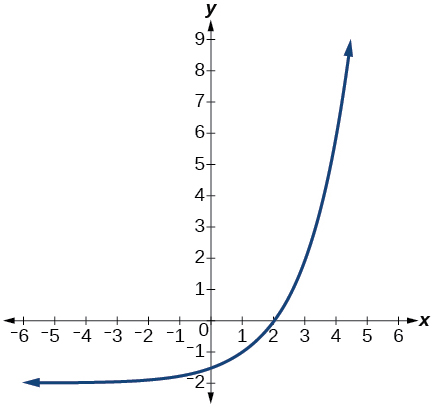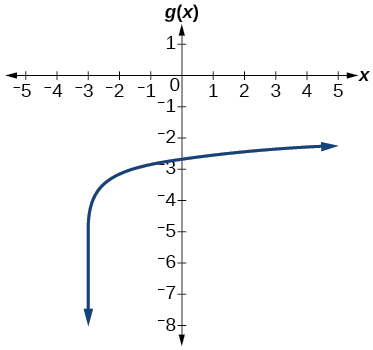# 6.8 Fitting exponential models to data  (Page 10/12)

 Page 10 / 12

The graph below shows transformations of the graph of $\text{\hspace{0.17em}}f\left(x\right)={2}^{x}.\text{\hspace{0.17em}}$ What is the equation for the transformation?## Logarithmic Functions

Rewrite $\text{\hspace{0.17em}}{\mathrm{log}}_{17}\left(4913\right)=x\text{\hspace{0.17em}}$ as an equivalent exponential equation.

${17}^{x}=4913$

Rewrite $\text{\hspace{0.17em}}\mathrm{ln}\left(s\right)=t\text{\hspace{0.17em}}$ as an equivalent exponential equation.

Rewrite $\text{\hspace{0.17em}}{a}^{-\text{\hspace{0.17em}}\frac{2}{5}}=b\text{\hspace{0.17em}}$ as an equivalent logarithmic equation.

${\mathrm{log}}_{a}b=-\frac{2}{5}$

Rewrite $\text{\hspace{0.17em}}{e}^{-3.5}=h\text{\hspace{0.17em}}$ as an equivalent logarithmic equation.

Solve for $\text{\hspace{0.17em}}x\text{\hspace{0.17em}}\text{\hspace{0.17em}}{\mathrm{log}}_{64}\left(x\right)=\frac{1}{3}\text{\hspace{0.17em}}$ to exponential form.

$x={64}^{\frac{1}{3}}=4$

Evaluate $\text{\hspace{0.17em}}{\mathrm{log}}_{5}\left(\frac{1}{125}\right)\text{\hspace{0.17em}}$ without using a calculator.

Evaluate $\text{\hspace{0.17em}}\mathrm{log}\left(\text{0}\text{.000001}\right)\text{\hspace{0.17em}}$ without using a calculator.

$\mathrm{log}\left(\text{0}\text{.000001}\right)=-6$

Evaluate $\text{\hspace{0.17em}}\mathrm{log}\left(4.005\right)\text{\hspace{0.17em}}$ using a calculator. Round to the nearest thousandth.

Evaluate $\text{\hspace{0.17em}}\mathrm{ln}\left({e}^{-0.8648}\right)\text{\hspace{0.17em}}$ without using a calculator.

$\mathrm{ln}\left({e}^{-0.8648}\right)=-0.8648$

Evaluate $\text{\hspace{0.17em}}\mathrm{ln}\left(\sqrt{18}\right)\text{\hspace{0.17em}}$ using a calculator. Round to the nearest thousandth.

## Graphs of Logarithmic Functions

Graph the function $\text{\hspace{0.17em}}g\left(x\right)=\mathrm{log}\left(7x+21\right)-4.$Graph the function $\text{\hspace{0.17em}}h\left(x\right)=2\mathrm{ln}\left(9-3x\right)+1.$

State the domain, vertical asymptote, and end behavior of the function $\text{\hspace{0.17em}}g\left(x\right)=\mathrm{ln}\left(4x+20\right)-17.$

Domain: $\text{\hspace{0.17em}}x>-5;\text{\hspace{0.17em}}$ Vertical asymptote: $\text{\hspace{0.17em}}x=-5;\text{\hspace{0.17em}}$ End behavior: as $\text{\hspace{0.17em}}x\to -{5}^{+},f\left(x\right)\to -\infty \text{\hspace{0.17em}}$ and as $\text{\hspace{0.17em}}x\to \infty ,f\left(x\right)\to \infty .$

## Logarithmic Properties

Rewrite $\text{\hspace{0.17em}}\mathrm{ln}\left(7r\cdot 11st\right)\text{\hspace{0.17em}}$ in expanded form.

Rewrite $\text{\hspace{0.17em}}{\mathrm{log}}_{8}\left(x\right)+{\mathrm{log}}_{8}\left(5\right)+{\mathrm{log}}_{8}\left(y\right)+{\mathrm{log}}_{8}\left(13\right)\text{\hspace{0.17em}}$ in compact form.

${\text{log}}_{8}\left(65xy\right)$

Rewrite $\text{\hspace{0.17em}}{\mathrm{log}}_{m}\left(\frac{67}{83}\right)\text{\hspace{0.17em}}$ in expanded form.

Rewrite $\text{\hspace{0.17em}}\mathrm{ln}\left(z\right)-\mathrm{ln}\left(x\right)-\mathrm{ln}\left(y\right)\text{\hspace{0.17em}}$ in compact form.

$\mathrm{ln}\left(\frac{z}{xy}\right)$

Rewrite $\text{\hspace{0.17em}}\mathrm{ln}\left(\frac{1}{{x}^{5}}\right)\text{\hspace{0.17em}}$ as a product.

Rewrite $\text{\hspace{0.17em}}-{\mathrm{log}}_{y}\left(\frac{1}{12}\right)\text{\hspace{0.17em}}$ as a single logarithm.

${\text{log}}_{y}\left(12\right)$

Use properties of logarithms to expand $\text{\hspace{0.17em}}\mathrm{log}\left(\frac{{r}^{2}{s}^{11}}{{t}^{14}}\right).$

Use properties of logarithms to expand $\text{\hspace{0.17em}}\mathrm{ln}\left(2b\sqrt{\frac{b+1}{b-1}}\right).$

$\mathrm{ln}\left(2\right)+\mathrm{ln}\left(b\right)+\frac{\mathrm{ln}\left(b+1\right)-\mathrm{ln}\left(b-1\right)}{2}$

Condense the expression $\text{\hspace{0.17em}}5\mathrm{ln}\left(b\right)+\mathrm{ln}\left(c\right)+\frac{\mathrm{ln}\left(4-a\right)}{2}\text{\hspace{0.17em}}$ to a single logarithm.

Condense the expression $\text{\hspace{0.17em}}3{\mathrm{log}}_{7}v+6{\mathrm{log}}_{7}w-\frac{{\mathrm{log}}_{7}u}{3}\text{\hspace{0.17em}}$ to a single logarithm.

${\mathrm{log}}_{7}\left(\frac{{v}^{3}{w}^{6}}{\sqrt{u}}\right)$

Rewrite $\text{\hspace{0.17em}}{\mathrm{log}}_{3}\left(12.75\right)\text{\hspace{0.17em}}$ to base $\text{\hspace{0.17em}}e.$

Rewrite $\text{\hspace{0.17em}}{5}^{12x-17}=125\text{\hspace{0.17em}}$ as a logarithm. Then apply the change of base formula to solve for $\text{\hspace{0.17em}}x\text{\hspace{0.17em}}$ using the common log. Round to the nearest thousandth.

$x=\frac{\frac{\mathrm{log}\left(125\right)}{\mathrm{log}\left(5\right)}+17}{12}=\frac{5}{3}$

## Exponential and Logarithmic Equations

Solve $\text{\hspace{0.17em}}{216}^{3x}\cdot {216}^{x}={36}^{3x+2}\text{\hspace{0.17em}}$ by rewriting each side with a common base.

Solve $\text{\hspace{0.17em}}\frac{125}{{\left(\frac{1}{625}\right)}^{-x-3}}={5}^{3}\text{\hspace{0.17em}}$ by rewriting each side with a common base.

$x=-3$

Use logarithms to find the exact solution for $\text{\hspace{0.17em}}7\cdot {17}^{-9x}-7=49.\text{\hspace{0.17em}}$ If there is no solution, write no solution .

Use logarithms to find the exact solution for $\text{\hspace{0.17em}}3{e}^{6n-2}+1=-60.\text{\hspace{0.17em}}$ If there is no solution, write no solution .

no solution

Find the exact solution for $\text{\hspace{0.17em}}5{e}^{3x}-4=6\text{\hspace{0.17em}}$ . If there is no solution, write no solution .

Find the exact solution for $\text{\hspace{0.17em}}2{e}^{5x-2}-9=-56.\text{\hspace{0.17em}}$ If there is no solution, write no solution .

no solution

Find the exact solution for $\text{\hspace{0.17em}}{5}^{2x-3}={7}^{x+1}.\text{\hspace{0.17em}}$ If there is no solution, write no solution .

Find the exact solution for $\text{\hspace{0.17em}}{e}^{2x}-{e}^{x}-110=0.\text{\hspace{0.17em}}$ If there is no solution, write no solution .

$x=\mathrm{ln}\left(11\right)$

Use the definition of a logarithm to solve. $\text{\hspace{0.17em}}-5{\mathrm{log}}_{7}\left(10n\right)=5.$

47. Use the definition of a logarithm to find the exact solution for $\text{\hspace{0.17em}}9+6\mathrm{ln}\left(a+3\right)=33.$

$a={e}^{4}-3$

Use the one-to-one property of logarithms to find an exact solution for $\text{\hspace{0.17em}}{\mathrm{log}}_{8}\left(7\right)+{\mathrm{log}}_{8}\left(-4x\right)={\mathrm{log}}_{8}\left(5\right).\text{\hspace{0.17em}}$ If there is no solution, write no solution .

Use the one-to-one property of logarithms to find an exact solution for $\text{\hspace{0.17em}}\mathrm{ln}\left(5\right)+\mathrm{ln}\left(5{x}^{2}-5\right)=\mathrm{ln}\left(56\right).\text{\hspace{0.17em}}$ If there is no solution, write no solution .

$x=±\frac{9}{5}$

The formula for measuring sound intensity in decibels $\text{\hspace{0.17em}}D\text{\hspace{0.17em}}$ is defined by the equation $\text{\hspace{0.17em}}D=10\mathrm{log}\left(\frac{I}{{I}_{0}}\right),$ where $\text{\hspace{0.17em}}I\text{\hspace{0.17em}}$ is the intensity of the sound in watts per square meter and $\text{\hspace{0.17em}}{I}_{0}={10}^{-12}\text{\hspace{0.17em}}$ is the lowest level of sound that the average person can hear. How many decibels are emitted from a large orchestra with a sound intensity of $\text{\hspace{0.17em}}6.3\cdot {10}^{-3}\text{\hspace{0.17em}}$ watts per square meter?

how do I set up the problem?
what is a solution set?
Harshika
hello, I am happy to help!
Abdullahi
find the value of 2x=32
divide by 2 on each side of the equal sign to solve for x
corri
X=16
Michael
Want to review on complex number 1.What are complex number 2.How to solve complex number problems.
Beyan
use the y -intercept and slope to sketch the graph of the equation y=6x
how do we prove the quadratic formular
hello, if you have a question about Algebra 2. I may be able to help. I am an Algebra 2 Teacher
thank you help me with how to prove the quadratic equation
Seidu
may God blessed u for that. Please I want u to help me in sets.
Opoku
what is math number
4
Trista
x-2y+3z=-3 2x-y+z=7 -x+3y-z=6
Need help solving this problem (2/7)^-2
x+2y-z=7
Sidiki
what is the coefficient of -4×
-1
Shedrak
the operation * is x * y =x + y/ 1+(x × y) show if the operation is commutative if x × y is not equal to -1
An investment account was opened with an initial deposit of \$9,600 and earns 7.4% interest, compounded continuously. How much will the account be worth after 15 years?
lim x to infinity e^1-e^-1/log(1+x)
given eccentricity and a point find the equiationByByBy Tamsin KnoxByBy Mucho MizindukoBy Yasser IbrahimBy OpenStaxBy RhodesBy Michael SagBy OpenStaxBy OpenStaxBy Abby Sharp